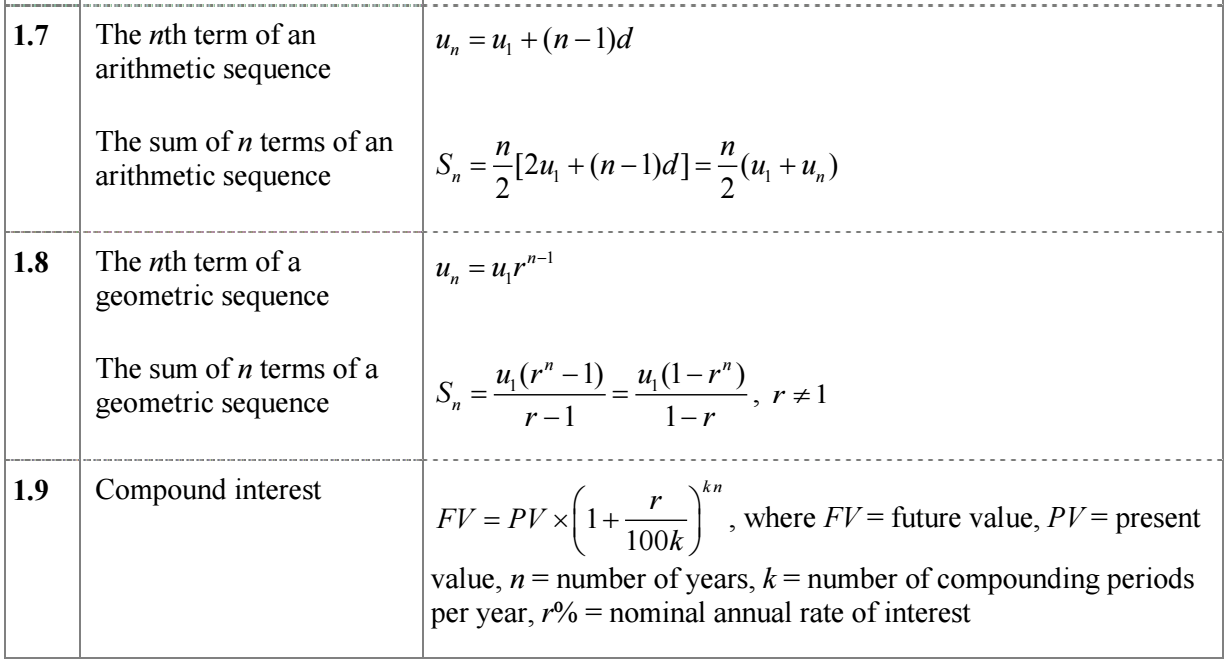### 11. Sequences, Series & Financial Mathematics

 Formula book:Textbook ReferenceChapter 7 p294-3277.1 Arithmetic Sequences p296-304- The sum of the first n terms of an arithmetic sequence- Applications of arithmetic sequences7.2 Geometric Sequences p304-309- Finding the nth term of a geometric sequence -The sum of the first n terms of a geometric sequence- Applications of geometric sequences 7.3 Currency Converions p310-314 7.4 Compound Interest p314-320-Annual depreciation and inflationReview exercises p321-324Chapter 7 Summary p325